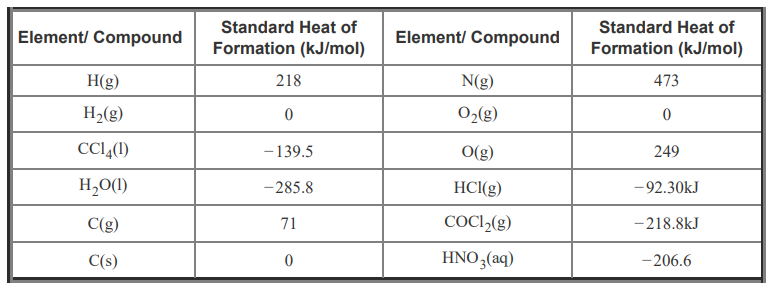# Problem: What is ΔH°rxn for the following chemical reaction? H2O(l) + CCl4(l) → COCl2(g) + 2 HCl(g) You can use the following table of standard heats of formation (ΔH°f ) to calculate the enthalpy of the given reaction.Express the standard enthalpy of reaction to three significant figures and include the appropriate units.The standard enthalpy of reaction is the enthalpy change that occurs in a reaction when all the reactants and products are in their standard states. The symbol for the standard enthalpy of reaction is ΔH°rxn, where the subscript "rxn" stands for "reaction." The standard enthalpy of a reaction is calculated from the standard heats of formation (ΔH°f ) (subscript "f" for formation) of its reactants and products. Therefore, the standard enthalpy ΔH°rxn of any reaction can be mathematically determined, as long as the standard heats of formation (ΔH°f) of its reactants and products are known.In a generic chemical reaction involving reactants A and B and products C and D, aA + bB → cC + dD, the standard enthalpy ΔH°rxn of the reaction is given byΔH°rxn = cΔH°f(C) + dΔH°f(D) − aΔH°f(A) − bΔH°f(B)Notice that the stoichiometric coefficients, a, b, c, d, are an important part of this equation. This formula is often generalized as follows, where the first sum on the right-hand side of the equation is a sum over the products and the second sum is over the reactants:ΔH°rxn = ΣproductsnΔH°f − ΣreactantsmΔH°fwhere m and n represent the appropriate stoichiometric coefficients for each substance.

###### FREE Expert SolutionView Complete Written Solution
###### Problem Details

What is ΔH°rxn for the following chemical reaction?

H2O(l) + CCl4(l) → COCl2(g) + 2 HCl(g)

You can use the following table of standard heats of formation (ΔH°f ) to calculate the enthalpy of the given reaction.Express the standard enthalpy of reaction to three significant figures and include the appropriate units.

The standard enthalpy of reaction is the enthalpy change that occurs in a reaction when all the reactants and products are in their standard states. The symbol for the standard enthalpy of reaction is ΔH°rxn, where the subscript "rxn" stands for "reaction." The standard enthalpy of a reaction is calculated from the standard heats of formation (ΔH°f ) (subscript "f" for formation) of its reactants and products. Therefore, the standard enthalpy ΔH°rxn of any reaction can be mathematically determined, as long as the standard heats of formation (ΔH°f) of its reactants and products are known.

In a generic chemical reaction involving reactants A and B and products C and D, aA + bB → cC + dD, the standard enthalpy ΔH°rxn of the reaction is given by

ΔH°rxn = cΔH°f(C) + dΔH°f(D) − aΔH°f(A) − bΔH°f(B)

Notice that the stoichiometric coefficients, a, b, c, d, are an important part of this equation. This formula is often generalized as follows, where the first sum on the right-hand side of the equation is a sum over the products and the second sum is over the reactants:

ΔH°rxn = ΣproductsnΔH°f − ΣreactantsmΔH°f

where m and n represent the appropriate stoichiometric coefficients for each substance.